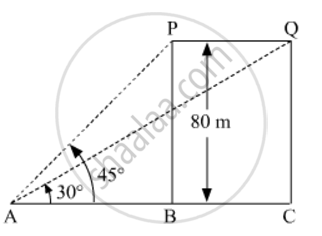# A bird is sitting on the top of a 80 m high tree. From a point on the ground, the angle of elevation of the bird is 45°. The bird flies away horizontally in such a way that it remained at a constant height from the ground. - Mathematics

A bird is sitting on the top of a 80 m high tree. From a point on the ground, the angle of elevation of the bird is 45°. The bird flies away horizontally in such a way that it remained at a constant height from the ground. After 2 seconds, the angle of elevation of the bird from the same point is 30°. Find the speed of flying of the bird.

("Take"sqrt3=1.732)

#### Solution

Let P and Q be the two positions of the bird, and let A be the point of observation. Let ABC be the horizontal line through A.

Given: The angles of elevations of the bird in two positions P and Q from point A are 45° and 30°, respectively.∴ ∠PAB = 45° and ∠QAB = 30°

Also,

PB = 80 m

In ΔABP, we have

tan45^@=(BP)/(AB)

=>1=80/(AB)

⇒ AB=80 m

In ΔACQ, we have

tan30^@=(CQ)/(AC)

=>1/sqrt3=80/(AC)

=>AC=800sqrt3 m

∴ PQ = BC = AC – AB = 80380=80 (sqrt31) m

So, the bird covers 80 (sqrt3- 1)min 2 s

Thus, speed of the bird is given by

=(80(sqrt3-1))/2

=(40(sqrt3-1)xx60xx60)/1000

=144(1.7321)km/h

=105.408 km/h

Concept: Heights and Distances
Is there an error in this question or solution?